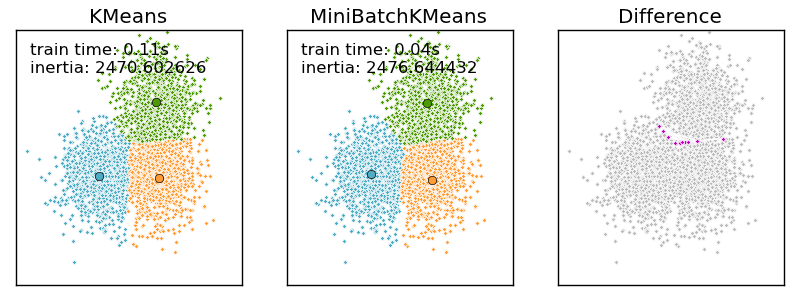This documentation is for scikit-learn version 0.11-gitOther versions

### Citing

If you use the software, please consider citing scikit-learn.

# A demo of the K Means clustering algorithm¶

We want to compare the performance of the MiniBatchKMeans and KMeans: the MiniBatchKMeans is faster, but gives slightly different results (see Mini Batch K-Means).

We will cluster a set of data, first with KMeans and then with MiniBatchKMeans, and plot the results. We will also plot the points that are labelled differently between the two algorithms.Python source code: plot_mini_batch_kmeans.py

```print __doc__

import time

import numpy as np
import pylab as pl

from sklearn.cluster import MiniBatchKMeans, KMeans
from sklearn.metrics.pairwise import euclidean_distances
from sklearn.datasets.samples_generator import make_blobs

##############################################################################
# Generate sample data
np.random.seed(0)

batch_size = 45
centers = [[1, 1], [-1, -1], [1, -1]]
n_clusters = len(centers)
X, labels_true = make_blobs(n_samples=3000, centers=centers, cluster_std=0.7)

##############################################################################
# Compute clustering with Means

k_means = KMeans(init='k-means++', k=3, n_init=10)
t0 = time.time()
k_means.fit(X)
t_batch = time.time() - t0
k_means_labels = k_means.labels_
k_means_cluster_centers = k_means.cluster_centers_
k_means_labels_unique = np.unique(k_means_labels)

##############################################################################
# Compute clustering with MiniBatchKMeans

mbk = MiniBatchKMeans(init='k-means++', k=3, batch_size=batch_size,
n_init=10, max_no_improvement=10, verbose=0)
t0 = time.time()
mbk.fit(X)
t_mini_batch = time.time() - t0
mbk_means_labels = mbk.labels_
mbk_means_cluster_centers = mbk.cluster_centers_
mbk_means_labels_unique = np.unique(mbk_means_labels)

##############################################################################
# Plot result

fig = pl.figure(figsize=(8, 3))
fig.subplots_adjust(left=0.02, right=0.98, bottom=0.05, top=0.9)
colors = ['#4EACC5', '#FF9C34', '#4E9A06']

# We want to have the same colors for the same cluster from the
# MiniBatchKMeans and the KMeans algorithm. Let's pair the cluster centers per
# closest one.

distance = euclidean_distances(k_means_cluster_centers,
mbk_means_cluster_centers,
squared=True)
order = distance.argmin(axis=1)

# KMeans
ax = fig.add_subplot(1, 3, 1)
for k, col in zip(range(n_clusters), colors):
my_members = k_means_labels == k
cluster_center = k_means_cluster_centers[k]
ax.plot(X[my_members, 0], X[my_members, 1], 'w',
markerfacecolor=col, marker='.')
ax.plot(cluster_center, cluster_center, 'o', markerfacecolor=col,
markeredgecolor='k', markersize=6)
ax.set_title('KMeans')
ax.set_xticks(())
ax.set_yticks(())
pl.text(-3.5, 1.8,  'train time: %.2fs\ninertia: %f' % (
t_batch, k_means.inertia_))

# MiniBatchKMeans
ax = fig.add_subplot(1, 3, 2)
for k, col in zip(range(n_clusters), colors):
my_members = mbk_means_labels == order[k]
cluster_center = mbk_means_cluster_centers[order[k]]
ax.plot(X[my_members, 0], X[my_members, 1], 'w',
markerfacecolor=col, marker='.')
ax.plot(cluster_center, cluster_center, 'o', markerfacecolor=col,
markeredgecolor='k', markersize=6)
ax.set_title('MiniBatchKMeans')
ax.set_xticks(())
ax.set_yticks(())
pl.text(-3.5, 1.8, 'train time: %.2fs\ninertia: %f' %
(t_mini_batch, mbk.inertia_))

# Initialise the different array to all False
different = (mbk_means_labels == 4)
ax = fig.add_subplot(1, 3, 3)

for l in range(n_clusters):
different += ((k_means_labels == k) != (mbk_means_labels == order[k]))

identic = np.logical_not(different)
ax.plot(X[identic, 0], X[identic, 1], 'w',
markerfacecolor='#bbbbbb', marker='.')
ax.plot(X[different, 0], X[different, 1], 'w',
markerfacecolor='m', marker='.')
ax.set_title('Difference')
ax.set_xticks(())
ax.set_yticks(())

pl.show()
```